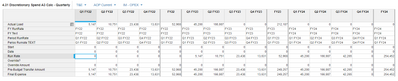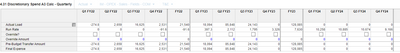# Average for a Period of Time for Next PeriodHi Team,

I am trying to calculate the "Average" for a Expense for the Period of "FY24". And I want to calculate using the Average from Q3 FY22 to Q2 FY23. On the "actual line items are the expenses for prior quarters and on "RunRate Line Item" I want to calculate the Average for those 4 prior quarters.

I am try TIME SUM and it didn't work unless I am missing something =( Would you please help me find a solution? Thanks!Yordana!

Tagged:

•Hi Yordana,

For moving time, to get the data of the last N, no of periods we cannot use TIMESUM. We need to use MOVINGSUM for calculating the data. In your case, you can apply the following formulae in Run rate to arrive at the solution.

Kindly let us know, if it worked out for you or not.

Thanks & Regards

Matasma Digital Technologies

•Your solution work but with a slightly change on the periods MOVINGSUM(Actual Load, -6, -3, AVERAGE).

That's the formula for Q1 FY24 but then I want to make Q2 FY24 to Q4 FY24 equals to Q1 FY24 because if not the formula for the other periods will take the zeros of the FY23 quarters that had not happened yet.

So on the example below - I will need that Q2 FY24 ---> have as value \$10,258.Thanks!

Yordana T.